# Processing：图像

1. 载入一张图片：
// 申明一个PImage类型的变量, 一个我们可用的存储于p5核心库内的类.
PImage img;

void setup() {
size(320,240);
// 通过载入一个图片文件创建一个新的PImage实例
img = loadImage(“fuckyoubaby.jpg”);
}

void draw() {
background(0);
//image()函数在一个位置显示图片-本例位于点(0,0).
image(img,0,0);
}

2. 在上例内放入一张比size大的图片，运行，它将以原尺寸从左上角开始显示图片，你的size有多大，他就显示多大，没有缩放。

3. 要显示的图片必须位于本sketch文件夹下的data文件夹内（这也是p5载入外部文件的统一规矩）。

4. 我们需要注意，从硬盘载入图片相对来说是较慢的，因此我们应当确保我们的程序仅执行其一次，因此，应当将它放到setup()而不是draw()里。

5. 一旦图片被载入，它将被image()函数显示出来，这个函数必备三个引数：被显示的图片、x位置和y位置，另外，后边还可接两个备选参数：图像的宽和高（全图缩放）。

6. 让图片动起来：
PImage dick; // 为图片文件准备的变量
float x,y; // 图片位置变量
float rot; // 图片旋转变量

void setup() {
size(200,200);

// 载入图像, 初始化变量
dick = loadImage(“fuckyoubaby.jpg”);
x = 0.0;
y = width/2.0;
rot = 0.0;
}

void draw() {
background(255);

// 位移和形变
translate(x,y);
rotate(rot);

// 图片可以像其他常规形状一样使用变量、translate(), rotate()等等进行动画制作.
image(dick,0,0);

// 调整动画变量
x += 1.0;
rot += 0.02;
if (x > width+head.width) {
x = -head.width;
}
}

7. 小丹出题：用类重写上例，是的，和你一样，目前为止我对类的使用还不是那么舒服，well，一起看答案吧，我始终坚信看着看着看多了也就会了。。

8. 如果你要改变图片显示的样子，tint()可以帮你，tint()之于图片就像fill()之于形状。tint()的引数可以简单指定每个像素可以使用的颜色数量以及透明度。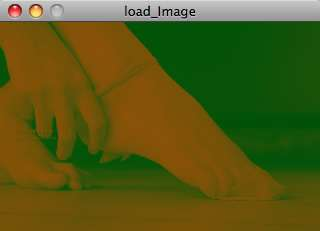tint()

◎ 如果tint()只接收到一个引数，只有图像的亮度会受到影响。
//原始亮度
tint(255);
image(sunflower,0,0);
//变暗
tint(100);
image(sunflower,0,0);
◎ 第二个引数将改变图片的alpha透明度
//设置图片不透明度为50%
tint(255,127);
image(sunflower,0,0);
◎ 三个引数影响红、绿、蓝三色组成的亮度。
//没有红色，大部分为绿色，全部为蓝色
tint(0,200,255)
image(sunflower,0,0);
◎ 最后，添加第四个引数同样控制alpha透明度。tint()值的范围可用colorMode()指定。
//设置图片为红色调并透明
tint(255,0,0,100);
image(sunflower,0,0);

9. 一张图片不爽，我们要一堆图片！我们从这个点子开始搞，载入五张图片，每点击鼠标一次更换一张。

PImage[] images = new PImage;

images = loadImage( ” cat.jpg ” );
images = loadImage( ” mouse.jpg ” );
images = loadImage( ” dog.jpg ” );
images = loadImage( ” kangaroo.jpg ” );
images = loadImage( ” porcupine.jpg ” );

String [] ﬁlenames = {“cat.jpg” , “mouse.jpg”, “dog.jpg” , “kangaroo.jpg” , “porcupine.jpg” );
for (int i = 0; i < ﬁlenames.length; i++) { images[i] = loadImage(ﬁlenames[i]); }

`知识串联：在字符串内：“Happy” ＋ “ Trails” ＝ “Happy Trails”，“2” ＋ “2” ＝ “22”。`

for (int i = 0; i < images.length; i++) { images[i] = loadImage( "animal" + i + ".jpg"); }

image(images,0,0);

image(images[imageindex],0,0);

int maxImages = 10; // 图片的总数
int imageIndex = 0; // 最初的图片最先显示

// 申明一个图片数组.
PImage[] images = new PImage[maxImages];

void setup() {
size(200,200);

// 将图片载入数组
// 别忘了将图片放入data文件夹!
for (int i = 0; i < images.length; i ++ ) { images[i] = loadImage( "animal" + i + ".jpg" ); } } void draw() { // 显示一副图 image(images[imageIndex],0,0); } void mousePressed() { // 当鼠标点击后随机选取一副图片显示 // 注意数组的索引必须是整数! imageIndex = int(random(images.length)); }

10. 顺序动态播放一组图片：
int maxImages = 10; // 图片的总数
int imageIndex = 0; // 最初的图片最先显示

// 申明一个图片数组.
PImage[] images = new PImage[maxImages];

void setup() {
size(200,200);

// 将图片载入数组
// 别忘了将图片放入data文件夹!
for (int i = 0; i < images.length; i ++ ) { images[i] = loadImage( "animal" + i + ".jpg" ); } frameRate(5); } void draw() { background(0); image(images[imageIndex],0,0); // 每次循环为索引加一 // 一旦到达数组末尾，使用模 " % "返回0 imageIndex = (imageIndex + 1) % images.length; }

11. 我们知道我们的萤幕是由像素组成的，我们之所以能用line()绘制两点间的直线，是因为p5为我们自动填满了这两点间所有像素的点。像素数组只有一维，从左之右、从上往下数序排列。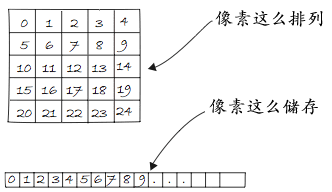像素布局

size(200,200);

// 在我们处理像素前
loadPixels();

// 在每个像素间循环
for (int i = 0; i < pixels.length; i++ ) { // 我们可以像处理其他数组那样得到像素数组的长度 // 选取一个随机数, 0到255 float rand = random(255); // 基于随机数创建一个灰色 color c = color(rand); // 为每个像素设置随机色 pixels[i] = c; // 我们可以通过索引进入像素数组的单独元素，与处理其他数组并无二致. } // 当我们完成像素处理工作 updatePixels();

12. 如上例，我们无需担心像素的具体位置，但是相素的XY位置在很多制图软件中是至关重要的信息。一个简单的例子：设置每个偶数列像素为白、每个奇数列像素为黑。通过一个一维像素数组你如何做到这一点？你如何知道任意给定的像素位于第几行第几列？当使用像素编程的时候，我们需要设想每个像素都存在于二维世界中，但是持续在一维接收数据。我们可以用如下公式：
1）假设一个窗口或图像大小为给定的宽度和高度。
2）于是我们知道了像素总数为宽*高。
3）对于任何给定的X、Y点，它在我们的一维像素数组中的位置是：位置＝X＋Y*宽度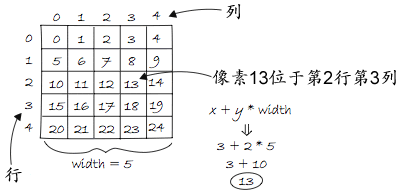像素位置及其x、y位置关系

13. 好的，现在让我们来处理上边那个奇数和偶数列的问题：
size(200,200);
loadPixels();

// 两个循环允许我们访问到每个列 (x) 和行 (y).

// 在每个像素列间循环
for (int x = 0; x < width; x++ ) { // 在每个像素行间循环 for (int y = 0; y < height; y++ ) { // 使用公式找到一维位置 int loc = x + y * width; if (x % 2 == 0) { // 如果我们位于偶数列 pixels[loc] = color(255); } else { // 如果我们位于奇数列 pixels[loc] = color(0); // 我们使用列数字 (x) 来决定颜色是黑是白 } } } updatePixels();

14. 这个练习的效果很有意思：
size(255, 255);
loadPixels();
for (int x = 0; x < width; x++) { for (int y = 0; y < height; y++) { int loc = x+y*width; float distance =dist(x,y,width/2,height/2); pixels[loc] = color(distance); } } updatePixels();

15. 还有这个：
size(255, 255);
loadPixels();
for (int x = 0; x < width; x++) { for (int y = 0; y < height; y++) { int loc = x + y*width; if (x < width/2) { pixels[loc] = color(x); } else { pixels[loc] = color(y); } } } updatePixels();

16. 之前的图像例子都是基于任意计算的像素值。现在我们将学习如何通过从一个已知PImage对象中找到的来设置像素，下边是一些伪码：
1）将一个图像载入PImage对象。
2）对于PImage中的每个像素，检索其颜色并用那个颜色颜色像素。
PImage包含很多有用的储存图像数据的域（field）——宽度、高度和像素，我们可以使用点语法（dot syntax）进入这些域：
PImage img = createImage(320,240,RGB); // 创建一个 PImage对象
println(img.width); // Yields 320
println(img.height); // Yields 240
img.pixels = color(255,0,0); // 设置第一个像素颜色为红色

17. 进入这些域允许我们循环一个图像的全部像素并将它们显示在萤幕上。
PImage img;

void setup() {
size(320 ,214);
img = loadImage(“fuckyoubaby.jpg”);
}

void draw() {
loadPixels();

// 由于要读取它的像素，我们必须同样在PImage呼叫loadPixels()
img.loadPixels();
for (int y = 0; y < height; y++ ) { for (int x = 0; x < width; x++ ) { int loc = x + y*width; // 函数red(), green(), and blue()将三个颜色的混合色显示在各个像素上. float r = red(img.pixels [loc]); float g = green(img.pixels[loc]); float b = blue(img.pixels[loc]); // 颜色处理将前往这里 // 如果我们想更改RGB值, 我们可以在这里做（在设置像素显示于萤幕前）. // 设置显示像素到图片像素上 pixels[loc] = color(r,g,b); } } updatePixels(); }

int imageLoc = x + y*img.width;
int displayLoc = x + y*width;

18. 是的，如果我们可以控制每个像素，也就意味着我们可以基于此玩出更多的把戏，请看下例，鼠标水平位置控制图像亮度:
PImage img;

void setup() {
size(320,214);
img = loadImage(“fuckyoubaby.jpg”);
}

void draw() {
loadPixels();

img.loadPixels();
for (int x = 0; x < img.width; x++ ) { for (int y = 0; y < img.height; y++ ) { // 计算1D像素位置 int loc = x + y*img.width; // 获取图像R,G,B值 float r = red (img.pixels[loc]); float g = green (img.pixels[loc]); float b = blue (img.pixels[loc]); // 用鼠标位置和乘法计算一个0.0到8.0的范围 // 这个乘法改变了每个像素的RGB值. float adjustBrightness = ((float) mouseX / width) * 8.0; r *= adjustBrightness; g *= adjustBrightness; b *= adjustBrightness; // 在设置为新颜色前，RGB值被限制在0到255间. r = constrain(r,0,255); g = constrain(g,0,255); b = constrain(b,0,255); // 制作一个新颜色并在窗口设置像素 color c = color(r,g,b); pixels[loc] = c; } } updatePixels(); }

19. 由于我们在每个像素的基础上改变图像，因此不需要平等对待每一像素。例如，我们可以根据其距离鼠标的距离改变每个像素的亮度：
PImage img;

void setup() {
size(320,214);
img = loadImage( “1.jpg” );
}

void draw() {
loadPixels();

// We must also call loadPixels() on the PImage since we are going to read its pixels. img.loadPixels();
for (int x = 0; x < img.width; x++ ) { for (int y = 0; y < img.height; y++ ) { // 计算1D像素位置 int loc = x + y*img.width; // 获取图像R,G,B值 float r = red (img.pixels[loc]); float g = green (img.pixels[loc]); float b = blue (img.pixels[loc]); // 基于与鼠标的接近度计算一个总量以改变亮度 float distance = dist(x,y,mouseX,mouseY); // 距离鼠标越近的像素, 其"distance"值越低 // 我们要更近的像素更亮, 因此我们将公式的值反向: float adjustBrightness = (50-distance)/50 // 距离鼠标50 (或更大)的像素的亮度为0.0 (或复数，这里为0) // 距离鼠标0的像素亮度为1.0. float adjustBrightness = (50-distance)/50; r *= adjustBrightness; g *= adjustBrightness; b *= adjustBrightness; // 将RGB限制在0～255 r = constrain(r,0,255); g = constrain(g,0,255); b = constrain(b,0,255); // 制作一个新颜色并在窗口设置像素 color c = color(r,g,b); pixels[loc] = c; } } updatePixels(); }

20. 我们之前的例子都是从原图读取每个像素并且只接在p5窗口内写下新像素。然而，我们常常使用一个更方便的方式将新的像素写到一个新的目标图像上（然后使用image()函数显示出来）。

21. 阀值（threshold）滤镜仅以两种状态显示一个图像的每个像素：黑或白（其实看下例，我们可以将它们设置为任意的两种颜色）。阀值可以自定义，如果像素的亮度高于阀值，则以白色来显示之，低于则用黑色。下例为阀值为100的情况：
PImage source; // 原图
PImage destination; // 目标图

void setup() {
size(200,200);
source = loadImage( “fuckyoubaby.jpg” );
//目标图被创建为一个与原图大小相当的空白图像
destination = createImage(source.width, source.height, RGB);
}

void draw() {
float threshold = 100;
// 我们将看一下全部图像的像素
source.loadPixels();
destination.loadPixels();

for (int x = 0; x < source.width; x++) { for (int y = 0; y < source.height; y++) { int loc = x + y*source.width; // 基于阀值比较亮度 // brightness()返回一个0～255间的值，像素颜色的整体亮度。 if (brightness(source.pixels[loc]) > threshold) {
destination.pixels[loc] = color(255); // 白
} else {
destination.pixels[loc] = color(0); // 黑
}
}
}
// 基于目标图更换像素
destination.updatePixels();
// 显示目标图
image(destination,0,0);
}

// 绘制图像
image(img,0,0);
// 使用阀值滤镜
// 0.5意味着阀值是亮度的50%
filter(THRESHOLD,0.5);

22. filter()函数提供一系列滤镜。这将改变图像显示的效果。模式除了THRESHOLD，还有GRAY, INVERT, POSTERIZE, BLUR, OPAQUE, ERODE 和 DILATE。参考p5官网看它们各自的例子。使用方法：
ﬁlter(mode);
ﬁlter(mode,level);

23. 之前我们的学习都是基于像素对像素，而为了进行更进一步的图像处理，我们必须进入像素组处理。

int loc = x + y*img.width;
color pix = img.pixels[loc];

int leftLoc = (x-1) + y*img.width;
color leftPix = img.pixels[leftLoc];

float diff = abs(brightness(pix) – brightness(leftPix));
pixels[loc] = color(diff);

// 由于我们要找的是左邻
// 因此我们跳过第一列
for (int x = 1; x < width; x++) { for (int y = 0; y < height; y++ ){ // 像素位置和颜色 int loc = x + y*img.width; color pix = img.pixels[loc]; // 左邻像素的位置和颜色 int leftLoc = (x –1) + y*img.width; color leftPix = img.pixels[leftLoc]; // 像素及其左邻的差是新颜色 float diff = abs(brightness(pix) - brightness(leftPix)); pixels[loc] = color(diff); } }

24. 上例我们查找两个像素找到边缘。然而更复杂的算法通常是寻找更多的相邻像素。总之，每个像素都有八个直接的邻居：上下左右，左上，左下，右上，右下。这些图形处理算法通常被称为“空间回旋（spatial convolution）”。处理使用一个输入像素及其相邻像素的加权平均计算一个输出像素。换句话说，那个新的像素是一个区域内像素的函数。可以使用不同的大小的相邻区域，比如3 × 3或5 × 5的矩阵等等。

–1 –1 –1
–1 9 –1
–1 –1 –1

1/9 1/9 1/9
1/9 1/9 1/9
1/9 1/9 1/9

25. 下例展示了一个使用2D数组存储一个3 × 3矩阵像素加权的回旋。这可能使我们到目前为止在本教程内碰到的最先进的例子，因为它使用了如此多的元素（嵌套循环，2D数组，PImage像素，等等），哦也，我看得也很崩溃，因为它太长了，放这慢慢研究吧…
PImage img;
int w = 80;
//一个”模糊”效果的回旋矩阵以一个3 x 3二维数组储存.
float[][] matrix = { { 1.0/9.0, 1.0/9.0, 1.0/9.0 } ,
{ 1.0/9.0, 1.0/9.0, 1.0/9.0 } ,
{ 1.0/9.0, 1.0/9.0, 1.0/9.0 } } ;

void setup() {
size(320,214);
img = loadImage( “fuckyoubaby.jpg” );
}

void draw() {

// 我们将仅处理图像的一部分
// 因此让我们将全图设为背景先
image(img,0,0);

// 在本例中我们仅仅处理图像的一部分—一个围绕鼠标的80 x 80矩形（鼠标分别向上下左右扩展40）.
int xstart = constrain(mouseX-w/2,0,img.width);
int ystart = constrain(mouseY-w/2,0,img.height);
int xend = constrain(mouseX + w/2,0,img.width);
int yend = constrain(mouseY + w/2,0,img.height);
int matrixsize = 3;

loadPixels();
// 为每个像素开始我们的循环
for (int x = xstart; x < xend; x++ ) { for (int y = ystart; y < yend; y++ ) { // 每个像素位置(x,y)开始进入一个名为convolution()的函数 // convolution()函数返回一个新的待显示的颜色. color c = convolution(x,y,matrix,matrixsize,img); int loc = x + y*img.width; pixels[loc] = c; } } updatePixels(); stroke(0); noFill(); rect(xstart,ystart,w,w); } color convolution(int x, int y, float[][] matrix, int matrixsize, PImage img) { float rtotal = 0.0; float gtotal = 0.0; float btotal = 0.0; int offset = matrixsize / 2; // 在回旋矩阵内循环 for (int i = 0; i < matrixsize; i++ ) { for (int j = 0; j < matrixsize; j++ ) { // 我们正在测试的像素是？ int xloc = x + i-offset; int yloc = y + j-offset; int loc = xloc + img.width*yloc; // 确保我们没有走出像素数组的边缘 // 在查找相邻像素时确保我们没有偶然走出像素数组的边缘常常是很好的。. loc = constrain(loc,0,img.pixels.length-1); // 计算回旋 // 我们通过乘以回旋矩阵的值以合计所有的相邻像素. rtotal += (red(img.pixels[loc]) * matrix[i][j]); gtotal += (green(img.pixels[loc]) * matrix[i][j]); btotal += (blue(img.pixels[loc]) * matrix[i][j]); } } // 确保RGB的范围 rtotal = constrain(rtotal,0,255); gtotal = constrain(gtotal,0,255); btotal = constrain(btotal,0,255); // 返回结果颜色 return color(rtotal,gtotal,btotal); }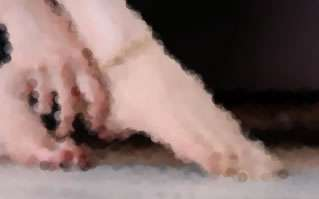随机点阵

26. 是的，你会和我有一样的疑问，上边这些难道PS不可以做么？为什么还要写这么大堆的代码？是的，它们只是帮助我们去理解做一些特效的原理，以及更底层的概念——像素。以下几个例子在PS里就很难完成了。抛开繁杂的编码，看下面这个绘制p5形状的算法。每循环一次draw()，我们以随机的位置画下一个圆，各个圆的颜色取自原图对应位置的颜色，来创建一种点阵图的特效。
PImage img;
int pointillize = 10;

void setup() {
size(320,214);
img = loadImage(“fuckyoubaby.jpg”);
background(255);
smooth();
}

void draw() {

// 选取一个随机点
int x = int(random(img.width));
int y = int(random(img.height));
int loc = x + y*img.width;

// 从原图查找RGB颜色
loadPixels();
float r = red(img.pixels[loc]);
float g = green(img.pixels[loc]);
float b = blue(img.pixels[loc]);

// 回到形状! 相对于设置一个像素, 我们使用一个像素的颜色来画一个圆.
noStroke();
fill(r,g,b,100);
ellipse(x,y,pointillize,pointillize);
}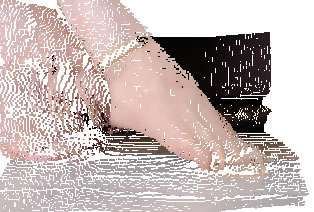2D转3D

27. 再看一个例子，我们从一个二维图像中获取数据，用3D位移技术在三维空间内的每一个像素渲染一个矩形。z的位置取决于颜色的亮度。越亮的看起来越靠近观者，越暗的则越远。
PImage img; // 原图
int cellsize = 2; // 点阵每个单元的尺寸
int cols, rows; // 我们系统中行和列的数目

void setup() {
size(320,214,P3D);
img = loadImage( “fuckyoubaby.jpg” ); // 载入图像
cols = width/cellsize; // 计算列的数目
rows = height/cellsize; // 计算行的数目
}

void draw() {
background(255);
img.loadPixels();

// 开始循环列
for (int i = 0; i < cols; i++ ) { // 开始循环行 for (int j = 0; j < rows; j++ ) { int x = i*cellsize + cellsize/2; // x位置 int y = j*cellsize + cellsize/2; // y位置 int loc = x + y*width; // 像素数组位置 color c = img.pixels[loc]; // 截取颜色 // 依mouseX和像素亮度计算z位置 float z = (mouseX/(float)width) * brightness(img.pixels[loc])- 100.0; // 位移到相应位置, 设置填充和边框, 并绘制矩形 pushMatrix(); translate(x,y,z); fill(c); noStroke(); rectMode(CENTER); rect(0,0,cellsize,cellsize); popMatrix(); } } }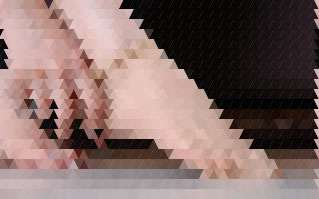三角三角！

28. 是的，可以用任何图形进行类似的填充，比如三角形：
PImage img;

void setup() {
size(320, 214);
// 载入图像
img = loadImage(“fuckyoubaby.jpg”);
smooth();
}

void draw() {
background(255);
// 处理像素前先呼叫loadPixels
loadPixels();
// 沿y轴每隔10个像素循环所有像素
for (int y = 0; y < img.height; y+=10 ) { // 沿x轴每隔5个像素循环所有像素 for (int x = 0; x < img.width+5; x+=5) { // 从一个2D网格计算1D位置 int loc = x + y*img.width; // 以原图填色 stroke(img.pixels[loc]); fill(img.pixels[loc]); // 顶点朝上或顶点朝下绘制三角形 if (x %10 == 0) triangle(x-5,y,x,y+10,x+5,y); else triangle(x-5,y+10,x,y,x+5,y+10); } } }

## 《Processing：图像》上有13条评论

1.yanxing说道：

非常喜欢你的博客！！！

Reply

2.戳子说道：

请问sketch文件夹的路径是什么？我没找到。。。。。。不好意思，问了个白痴问题，我是计算机盲，刚开始学processing。

Replyww Reply:

比如说你新建一个文件，这个文件类型在processing中叫做sketch，你编辑完成后保存它为image.pde，processing会在大的sketchbook文件夹（位置在设置里指定）你指定的位置自动将其保存在一个名为image的文件夹内，我文中提到的“sketch文件夹”即这个名为image的文件夹。

要显示一张图片，你必须在image文件夹内，手动新建一个名为“data”的文件夹，然后将你欲显示的图片放入data文件夹内，再在imag.pde内写入正确的代码（如文中所示），既可以顺利运行显示图片。

如果你是一名初学者，建议你从第一课开始学起，搞清楚一些基础性的概念是很重要的。欲速则不达。你可以在网站地图页面按章节顺序学习。

Reply

3.戳子说道：

非常感谢，你的解释很详细。的确是我太着急了，应该一步一步来，你的笔记对我来说非常有用，我一直都在看，基本上很多东西都是看你的笔记开始做的。
请继续更新，我会一直关注，加油！

Reply

4.chris说道：

很浅显易懂。谢谢

想问问，如果我想把旋转的图片，MAP到一个固定的形状（比如三角形），是使用texture()函数么？

：）

Replyww Reply:

是的。但要注意，texture()函数必须在beginShape()和endShape()之间、vertex()之前被调用。

Reply

5.eva说道：

老板让我用pocessing做一个关于多点触摸的项目，电子翻书类，你有这个相关的资料吗？发个网址给我也好，谢谢谢谢！

Replyww Reply:

对不起，完全没有了解

Reply

6.eva说道：

就是 multi-touch pocessing,有研究的话带带我

Reply

7.kexike说道：

请问写了这些程序后如何导出PDF，或者可以印刷的大图呢？

Replyww1way Reply:

Reply

8.加一说道：

不知道大佬还会不会看，想知道怎么才能让图片镜像变换，网上实在找不到了，如果大佬看见了能解答一下吗？？万分感谢

Replyww1way Reply:

@加一, 什么叫做“图片镜像变换”？

Reply